# ISODD Excel FunctionArticle byExcelMojo Team## What Is ISODD Function In Excel?

The ISODD function in Excel identifies the odd numbers and returns TRUE if found, else returns FALSE. The function detects an odd number as a cell value or the result of a formula while performing mathematical calculations.

The ISODD Excel function is an inbuilt Information function, so we can insert the formula from the “Function Library” or enter it directly in the worksheet.

For example, we will determine if the numbers are odd values and display the result as logical values, TRUE or FALSE.

The table below contains a list of numbers.

Select cell B2, enter the formula =ISODD(A2), press “Enter”, and drag the formula from cell B2 to B7 using the fill handle. The output is shown below. Column C is for our reference.

[Output Observation: For instance, cells A2 and A3, contain odd numbers. And hence, the ISODD () in the corresponding target cells returns TRUE as the output. In the case of row 6, cell A6 contains a decimal value of 3.2. And the ISODD () truncates the decimal value, checks whether the truncated value is odd, and returns the output accordingly. So, it truncates 3.2 to 3. And as the value three is odd, the function output in the target cell B6 is TRUE.

On the other hand, as cells A4 and A5 contain even numbers, the function output in the target cells B4:B5 is FALSE. And the ISODD () return value is FALSE in cell B5 because 0 is an even number.

Next, in cell A7 is 5/11. When the supplied number is a fraction, the function considers its decimal equivalent and then processes the decimal value as explained above. So, the ISODD function checks if its decimal equivalent, 0.454545, is odd. It truncates it to 0. And as 0 is an even number, the function returns FALSE as the output.]

###### Key Takeaways
• The ISODD function in Excel checks if the given number is odd, with the return value always being a logical value, either TRUE if the number is odd or FALSE for an even value.
• The function accepts one mandatory argument, number, which can be the cell reference to the number, or a formula returning a numeric value.
• We can use the function with other Excel functions such as IF(), MOD(), SUM(), and Conditional Formatting to highlight the required results.
• Application.WorksheetFunction.ISODD(Arg1) is the Excel VBA ISODD method, where the Arg1 argument value is the number we require to check if it is odd.

### ISODD() Excel Formula

The syntax of the ISODD Excel formula is,

The argument of the ISODD Excel formula is,

• number: The dataset to test whether the numeric values are odd. It is a mandatory argument.

### How To Use ISODD Excel Function?

We can use the ISODD function in 2 ways, namely,

1. Access from the Excel ribbon.
2. Enter in the worksheet manually.

#### Method #1 – Access from the Excel ribbon

Choose an empty cell for the output → select the “Formulas” tab → go to the “Function Library” group → click the “More Functions” options drop-down → click the “Information” option right arrow → select the “ISODD” function, as shown below.

The “Function Arguments” window appears. Enter the argument in the “Number” field → click “OK”, as shown below.

#### Method #2 – Enter in the worksheet manually

The steps to use the ISODD Excel function are as follows:

1. Select an empty cell for the output.
2. Type =ISODD( in the cell. [Alternatively, type =I or =IS and double-click the ISODD function from the Excel suggestions.]
3. Enter the arguments as cell values or excel cell references and close the brackets.
4. Press Enter to view the outcome.

We will determine if the dataset contains even values using ISODD function.

The below table contains a list of values and their descriptions.

The steps to find the odd numbers using ISODD() are,

1. Select cell C2, and enter the formula =ISODD(A2).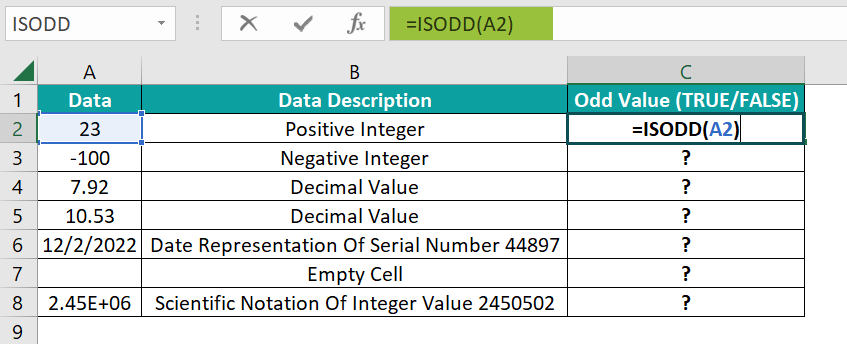2. Press the Enter. The result is “TRUE”, as shown below.

[Note: We can use alternate formulas such as =ISODD(23) or =ISODD(“23”) in the target cells to get the same results.]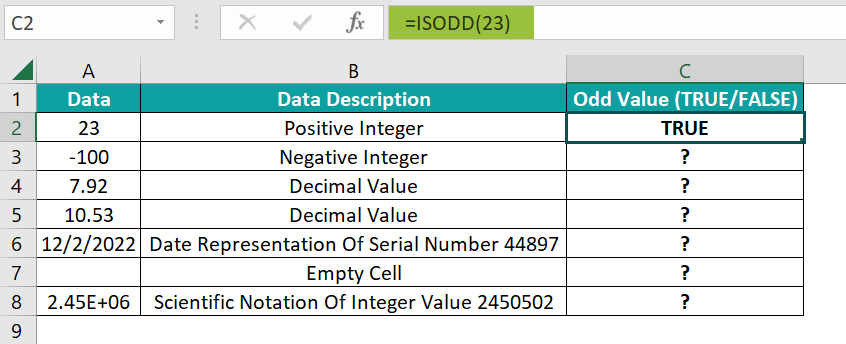3. Drag the formula from cell C2 to C8 using the fill handle. The output is shown below.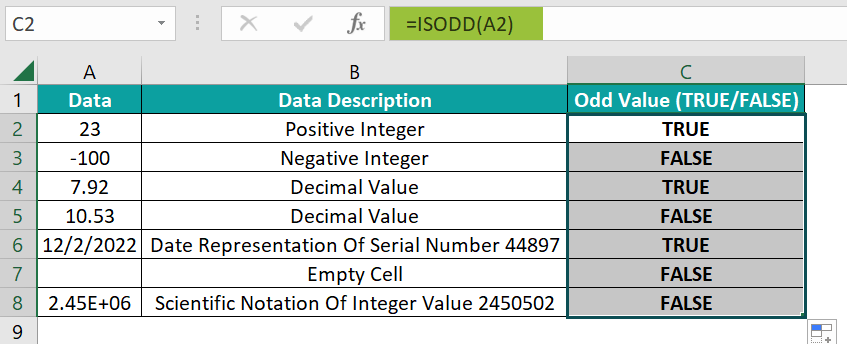[Alternatively, choosing the target cell and selecting FormulasMore FunctionsInformationISODD will apply the ISODD() in the selected cell from the Formulas tab.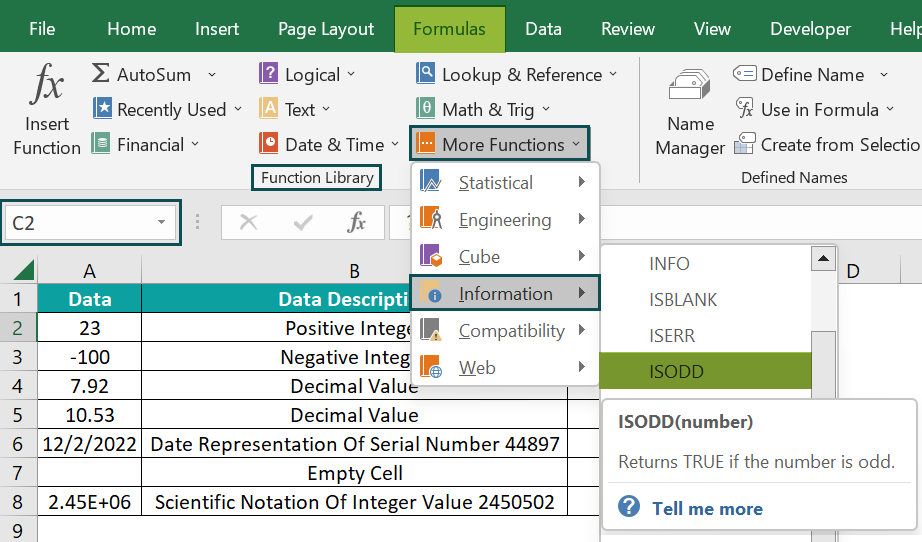Following the above step opens the Function Arguments window, where we enter the specified number or cell reference to the number. Now, click OK to close the Function Arguments window, and we will see the ISODD() output in cell C2.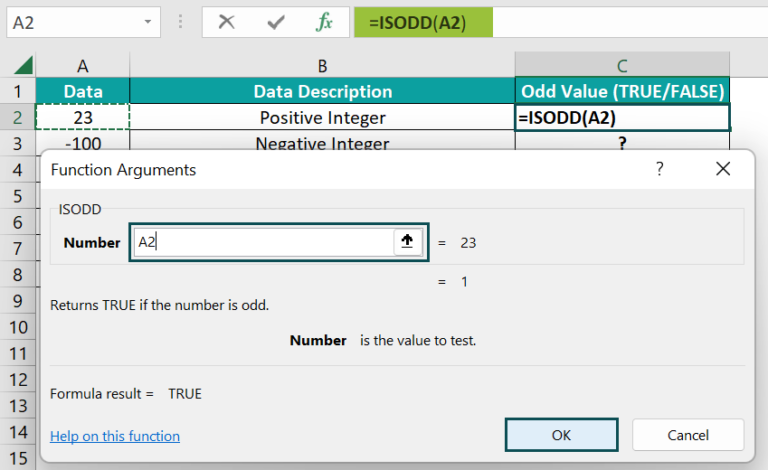[Output Observation: Cells A2 & A3 contain positive and negative integers. Cell A2 value is odd, and A3 is even, the ISODD() in the target cells C2 & C3 returns TRUE and FALSE, respectively.

Cells A4 & A5 contain decimal values. So, the ISODD() in the respective target cells, C4 & C5, truncates the decimal values to 7 and 10, respectively. And as seven is odd and 10 is an even value, the function in cells C4 & C5 returns TRUE and FALSE as the outcomes.

On the other hand, cell A6 contains the date representation of the serial number 44897. The ISODD() converts the date into its serial number equivalent and checks if it is odd. And as the resulting serial number, 44897, is odd, the function returns TRUE.

Next, the supplied ISODD function argument in cell C7 is a blank cell. The function considers it as 0. And as 0 is an even number, the function output is FALSE.

Finally, cell A8 shows the scientific notation of the integer 2450502. So, the ISODD() checks whether the integer is odd. And as the specified value is even, the function return value is FALSE.]

### Examples

We will understand some advanced scenarios using the ISODD function examples.

#### Example #1

We will find the total supply of each fruit received from 4 suppliers. And then, divide each fruit equally into boxes containing 100 units, and show if the fruits require an odd number of boxes using the ISODD function.

The below table contains a list of fruits and their supply data.

The steps to find the odd numbers using ISODD() and SUM() functions are,

• Step 1: Select the target cell F2, enter the formula =ISODD (SUM(B2:E2)/100), and press Enter. The result is ‘False’, as shown below.
• Step 2: Drag the formula from cell F2 to F6 using the fill handle. The output is shown below.

[Output Observation: In cell F6, first, the SUM() returns the sum of values in cells B6:E6, 503. Next, the expression inside the ISODD function divides the sum value of 503 by 100 to return a value of 5.03. Finally, the ISODD function truncates the value 5.03 to 5. And as 5 is an odd value, the function returns TRUE as the output.]

#### Example #2

We will determine the given number modulo 2 in each row, and check if the remainder is odd or even using the ISODD(), MOD(), and IF() functions.

The below table contains a list of numbers.

The steps to find the odd numbers using ISODD(), MOD(), and IF() functions are,

• Step 1: Select cell B2, enter the formula=IF(ISODD(MOD(A2,2)),”Odd Remainder”,”Even Remainder”), and press Enter. The result is “Even Remainder”, as shown below.
• Step 2: Drag the formula from cell B2 to B6 using the fill handle. The output is shown below.

[Output Observation: In cell B6, first, the MOD() determines -1559 mod 2, 1. Next, the ISODD  function, which is the IF condition, checks if the MOD() output is odd. As 1 is an odd number, the ISODD function returns TRUE.

Thus, as the IF condition holds in cell B6, the IF() returns Odd Remainder as the required output.]

#### Example #3

In the given dataset, we will highlight the odd number of units delivered using the ISODD() and the Conditional Formatting.

The following table contains a list of laptops and their units’ delivery details for April by five dealers.

The steps to find the odd numbers using ISODD() and Conditional Formatting are,

• 1: Select the cell range B3:F9 and go to HomeConditional FormattingNew Rule.

The New Formatting Rule window opens, as shown below.

• 2: In the “Select a Rule Type:” group, choose the “Use a formula to determine which cells to format” option, as shown below.
• 3: Now, in the “Edit the Rule Description:” group, enter the formula =ISODD(B3), and click the “Format” button, as shown below.

This step will open the Format Cells window, as shown below.

• 4: Now, select the Fill tab, pick a color to highlight the qualifying row cells, and click OK to close the Format Cells window.
• 5: Click OK in the New Formatting Rule window to close it and view the highlighted rows in the source table.

We will get the following output.

[Output Observation: The ISODD function applied as the Conditional Formatting rule checks every cell in the cell range B3:F9 whether the specified value is odd. And if the ISODD function output is TRUE for a specific cell, the cell gets highlighted in the selected color.]

### Important Things To Note

• If the specified value is a fraction, Excel converts it into a decimal, truncates it, checks if the truncated value is even, and returns the appropriate logical value.
• If the supplied argument is non-numeric, the function return value will be the #VALUE! error.
• When the cell value is a blank cell, the function considers it as 0, by default. And as 0 is an even number, the function output is FALSE.

1. Is there an ISODD function in Excel?

There is an ISODD function in Excel in the Formulas tab. To apply the function in a cell, select the cell and go to FormulasMore FunctionsInformationISODD.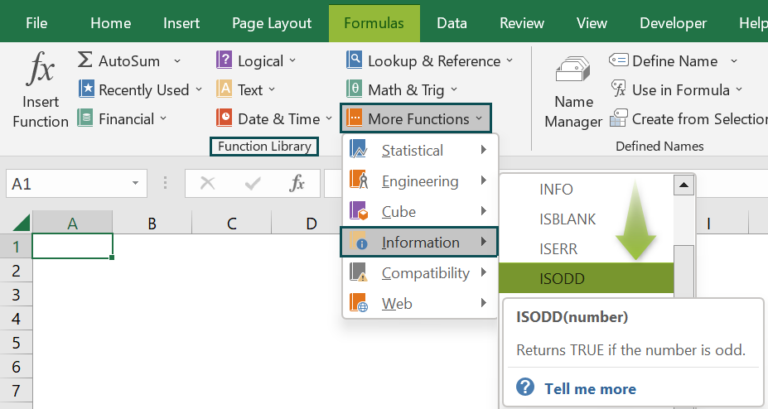2. How to apply the ISODD function in Excel VBA?

We can apply the ISODD function in Excel VBA using the method:

Application.WorksheetFunction.ISODD (Arg1)

For instance, we will find whether the listed numbers are odd using the ISODD and the Excel VBA.

The below table shows a list of numeric values.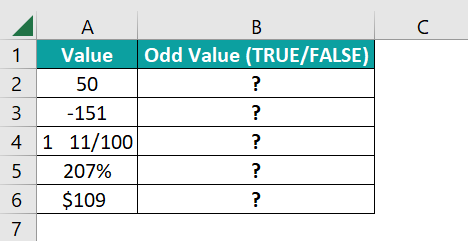The steps to find the odd numbers using the ISODD in Excel VBA are,

• 1: Open the worksheet containing the above table and press Alt + F11 to open the VBA Editor.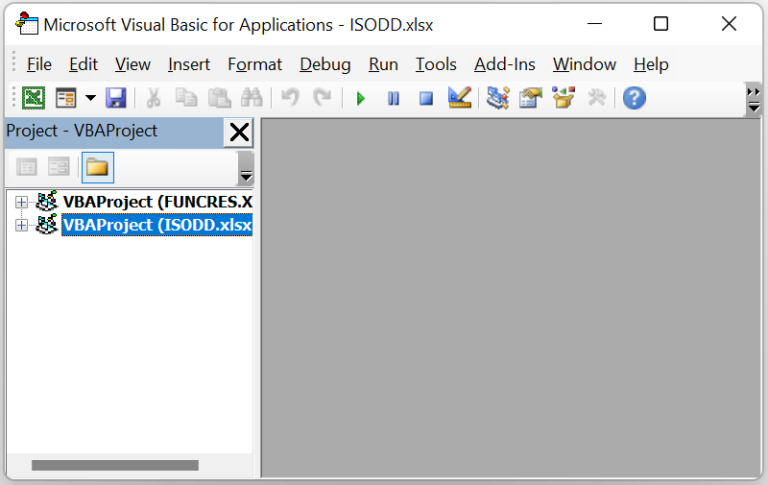• 2: Select the required VBAProject and choose the Module option from the Insert tab to open a new module window.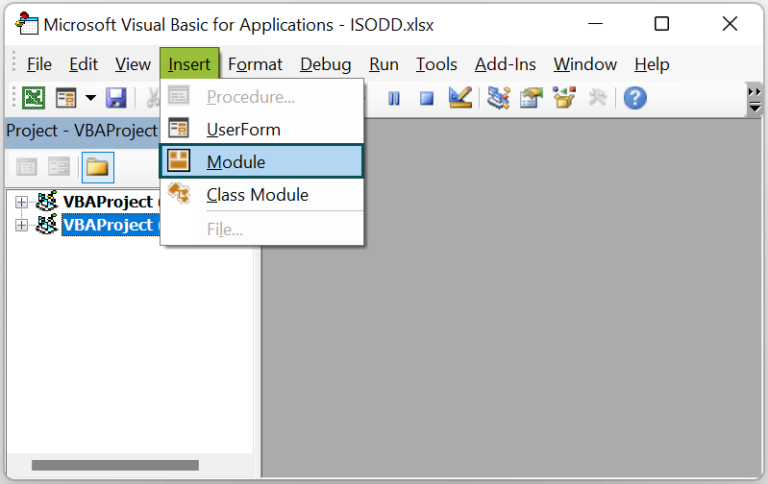• 3: Enter the VBA code, shown below, to apply the ISODD() in the target cells.

Sub ISODD_fn()
Dim ws As Worksheet
Set ws = Worksheets(“FAQ_2”)
ws.Range(“B2”) = Application.WorksheetFunction.IsOdd(ws.Range(“A2”))
ws.Range(“B3”) = Application.WorksheetFunction.IsOdd(ws.Range(“A3”))
ws.Range(“B4”) = Application.WorksheetFunction.IsOdd(ws.Range(“A4”))
ws.Range(“B5”) = Application.WorksheetFunction.IsOdd(ws.Range(“A5”))
ws.Range(“B6”) = Application.WorksheetFunction.IsOdd(ws.Range(“A6”))
End Sub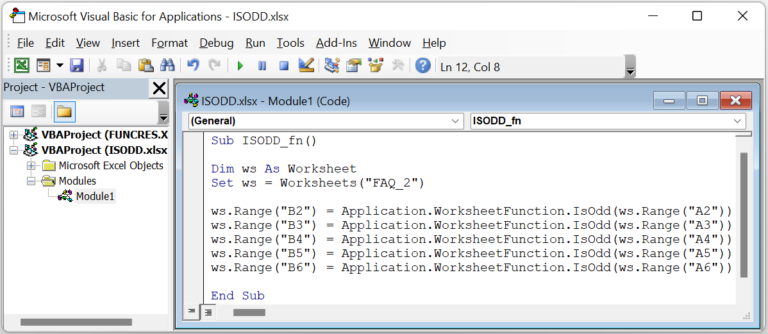• 4: Click the Run Sub/UserForm icon to execute the code.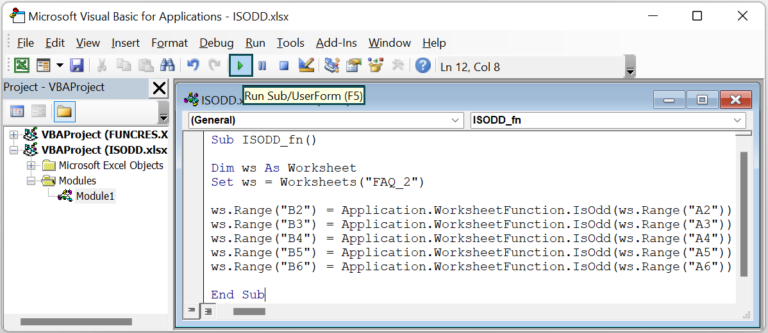Finally, open the active worksheet to view the result in the target cells.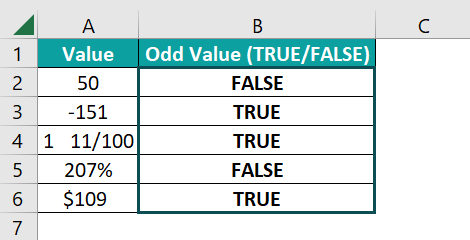[Output Observation: Cells A2:A3 contain positive and negative integers. The ISODD() in the target cells B2:B3 checks the odd values and returns FALSE and TRUE, as the cell A2 value is even and the cell A3 value is odd.

Cell A4 contains a fractional number 1. The ISODD() checks its decimal equivalent, 1.11, whether it is odd. So, the function truncates the decimal value to 1, and as 1 is an odd number, the function returns TRUE.

In the case of row 5, the ISODD() considers the decimal equivalent of the given cell A5 value of 207%, 2.07, to determine if the specified value is odd. Thus, the function truncates the decimal value to 2. And as 2 is an even number, the function returns FALSE.

On the other hand, cell A6 shows a currency value of \$109. And as the specified value is odd, the ISODD() returns TRUE.]

3. Why is the ISODD function not working in Excel?

The ISODD function may not work due to the following reasons.
We provided a non-numeric value as the function argument.
We applied the ISODD() as an array formula.